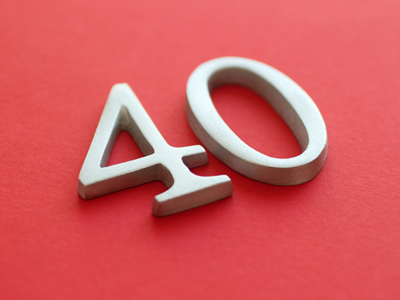4 × 10 = 40.

# 4 Times Table

Times tables are an important part of 11-Plus maths. One way of learning them is to practise them over and over again until you know them by heart. This will help you in later life when you will have to multiply numbers more often than you think!

The four times table means multiplying numbers by 4, or quadrupling them. Four is a number you'll encounter often. There are four suits in a deck of cards: hearts, diamonds, spades and clubs. Geographical directions are based on four key compass directions: north, south, east and west. There are four key phases of the moon: full moon, first quarter, new moon and third quarter. The year has four seasons: spring, summer, autumn and winter. There are four wings on a bee. Four is a very common number indeed.

You might think that you know your four times table - but be careful! Make sure you read every question properly and don't click on a wrong answer by mistake! Good luck.

1.
4 × 11 = ?
44
48
40
45
To calculate this answer you could first multiply 4 x 10 and then add 4
2.
4 × 12 = ?
16
32
43
48
There are 48 hours in 2 days
3.
4 × 3 = ?
15
18
12
9
A polyhedron with 12 faces is called a dodecahedron
4.
4 × 7 = ?
24
26
28
32
To multiply a number by 4, double it and then double your answer
5.
4 × 8 = ?
28
32
36
24
Water freezes at 32 degrees Fahrenheit
6.
4 × 5 = ?
20
15
24
25
To times a number by 5, first multiply it by 10 and then halve your answer
7.
4 × 6 = ?
20
28
24
16
There are 24 points on a backgammon board
8.
4 × 4 = ?
18
12
20
16
When we multiply a number by itself we square it. 16 is 4 squared
9.
4 × 9 = ?
36
32
34
38
To work this one out multiply 4 x 10 and then subtract 4
10.
4 × 2 = ?
6
8
4
12
A polygon with 8 sides is an octagon. 'Oct' means 8 in Latin
Author:  Frank Evans